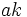# Join-transitively subnormal subgroup

## Definition

### Symbol-free definition

A subgroup of a group is termed join-transitively subnormal if its join (viz., the subgroup generated) with any subnormal subgroup is again subnormal.

### Definition with symbols

A subgroup$H$ of a group$G$ is termed join-transitively subnormal if whenever$K \triangleleft \triangleleft G$ (viz.,$K$ is subnormal in$G$), the join of subgroups$\langle H,K \rangle$ is subnormal in$G$.

BEWARE! This term is nonstandard and is being used locally within the wiki. [SHOW MORE]
This article defines a subgroup property: a property that can be evaluated to true/false given a group and a subgroup thereof, invariant under subgroup equivalence. View a complete list of subgroup properties[SHOW MORE]
If the ambient group is a finite group, this property is equivalent to the property: subnormal subgroup
View other properties finitarily equivalent to subnormal subgroup | View other variations of subnormal subgroup |

## Formalisms

### In terms of the join-transiter

This property is obtained by applying the join-transiter to the property: subnormal subgroup
View other properties obtained by applying the join-transiter

The subgroup property of being join-transitively subnormal is obtained by applying the join-transiter to the subgroup property of being subnormal.

## Relation with other properties

### Stronger properties

Property Meaning Proof of implication Proof of strictness (reverse implication failure) Intermediate notions
Normal subgroup Normal implies join-transitively subnormal Follows from 2-subnormal implies join-transitively subnormal, 2-subnormal not implies normal 2-subnormal subgroup, Asymptotically fixed-depth join-transitively subnormal subgroup, Conjugate-join-closed subnormal subgroup, Intermediately join-transitively subnormal subgroup, Join of finitely many 2-subnormal subgroups, Join-transitively 2-subnormal subgroup, Linear-bound join-transitively subnormal subgroup, Permutable subnormal subgroup, Polynomial-bound join-transitively subnormal subgroup, Subnormal-permutable subnormal subgroup|FULL LIST, MORE INFO
2-subnormal subgroup Normal subgroup of normal subgroup 2-subnormal implies join-transitively subnormal Follows from finite implies subnormal join property, and there exist subgroups of arbitrarily large subnormal depth in finite groups Conjugate-join-closed subnormal subgroup, Intermediately join-transitively subnormal subgroup, Join of finitely many 2-subnormal subgroups, Linear-bound join-transitively subnormal subgroup, Polynomial-bound join-transitively subnormal subgroup|FULL LIST, MORE INFO
Linear-bound join-transitively subnormal subgroup There's a constant$a$ such that the join with any$k$-subnormal subgroup is subnormal of depth at most$ak$ (by definition)  ? Polynomial-bound join-transitively subnormal subgroup|FULL LIST, MORE INFO
Polynomial-bound join-transitively subnormal subgroup There's a polynomial function$p$ such that the join with any$k$-subnormal subgroup is subnormal of depth at most$p(k)$ (by definition)  ? |FULL LIST, MORE INFO
Subnormal-permutable subnormal subgroup subnormal and permutes with all subnormal subgroups Subnormal-permutable and subnormal implies join-transitively subnormal finite implies subnormal join property, and examples of subnormal subgroups of finite groups that are not subnormal-permutable Intermediately join-transitively subnormal subgroup, Polynomial-bound join-transitively subnormal subgroup|FULL LIST, MORE INFO
Permutable subnormal subgroup subnormal and a permutable subgroup of the whole group Permutable and subnormal implies join-transitively subnormal (via subnormal-permutable subnormal) Intermediately join-transitively subnormal subgroup|FULL LIST, MORE INFO
Perfect subnormal subgroup subnormal subgroup and also a perfect group Perfect subnormal implies join-transitively subnormal normal implies join-transitively subnormal, examples of normal subgroups that are not perfect Intermediately join-transitively subnormal subgroup, Subnormal-permutable subnormal subgroup|FULL LIST, MORE INFO
Subnormal subgroup of finite index subnormal subgroup that is also a subgroup of finite index Subnormal of finite index implies join-transitively subnormal Asymptotically fixed-depth join-transitively subnormal subgroup, Conjugate-join-closed subnormal subgroup, Intermediately join-transitively subnormal subgroup, Linear-bound join-transitively subnormal subgroup|FULL LIST, MORE INFO
Subnormal subgroup of finite group subnormal subgroup of finite group Finite implies subnormal join property Asymptotically fixed-depth join-transitively subnormal subgroup, Intermediately join-transitively subnormal subgroup|FULL LIST, MORE INFO
Conjugate-join-closed subnormal subgroup join of any collection of its conjugate subgroups is subnormal Conjugate-join-closed subnormal implies join-transitively subnormal Intermediately join-transitively subnormal subgroup|FULL LIST, MORE INFO
Automorph-join-closed subnormal subgroup join of any collection of its automorphic subgroups is subnormal (via conjugate-join-closed) Intermediately join-transitively subnormal subgroup|FULL LIST, MORE INFO
Intermediately join-transitively subnormal subgroup join-transitively subnormal in every intermediate subgroup (by definition) |FULL LIST, MORE INFO

### Weaker properties

Property Meaning Proof of implication Proof of strictness (reverse implication failure) Intermediate notions
Finite-automorph-join-closed subnormal subgroup Join-transitively subnormal implies finite-automorph-join-closed subnormal |FULL LIST, MORE INFO
Finite-conjugate-join-closed subnormal subgroup (via finite-automorph-join-closed subnormal subgroup) Finite-automorph-join-closed subnormal subgroup|FULL LIST, MORE INFO

## Metaproperties

Metaproperty name Satisfied? Proof Statement with symbols
Transitive subgroup property No normal implies join-transitively subnormal, subnormality is not finite-join-closed We can have$H \le K \le G$ such that$H$ is join-transitively subnormal in$K$ and$K$ is join-transitively subnormal in$G$, but$H$ is not join-transitively subnormal in$G$.
Trim subgroup property Yes Every group is join-transitively subnormal in itself; the trivial subgroup is join-transitively subnormal.
Finite-intersection-closed subgroup property Known open problem intersection problem for join-transitively subnormal subgroups Given join-transitively subnormal subgroups$H,K$ of a group$G$, is$H \cap K$ necessarily join-transitively subnormal?
Intermediate subgroup condition Possibly open problem (see intermediately join-transitively subnormal subgroup) If$H \le K \le G$ such that$H$ is join-transitively subnormal in$G$, is$H$ necessarily join-transitively subnormal in$K$.
Finite-join-closed subgroup property Yes If$H, K \le G$ are both join-transitively subnormal in$G$, then$\langle H, K \rangle$ is also join-transitively subnormal.

### Trimness

This subgroup property is trim -- it is both trivially true (true for the trivial subgroup) and identity-true (true for a group as a subgroup of itself).
View other trim subgroup properties | View other trivially true subgroup properties | View other identity-true subgroup properties

Clearly, the whole group is join-transitively subnormal, because its join with any subgroup is the whole group. Also, the trivial subgroup is join-transitively subnormal, because its join with any subnormal subgroup is the same subnormal subgroup.

### Join-closedness

YES: This subgroup property is join-closed: an arbitrary (nonempty) join of subgroups with this property, also has this property.
ABOUT THIS PROPERTY: View variations of this property that are join-closed | View variations of this property that are not join-closed
ABOUT JOIN-CLOSEDNESS: View all join-closed subgroup properties (or, strongly join-closed properties) | View all subgroup properties that are not join-closed | Read a survey article on proving join-closedness | Read a survey article on disproving join-closedness

By the general theory of transiters, the join-transiter of any subgroup property is itself a finite-join-closed subgroup property.